# NCERT Solutions for Class 6 Maths Chapter 10: Mensuration Exercise 10.1

In this page we have NCERT Solutions for Class 6 Maths Chapter 10: Mensuration Exercise 10.1. Hope you like them and do not forget to like , social share and comment at the end of the page.

## Exercise 10.1

Question 1
Find the perimeter of each of the following figures: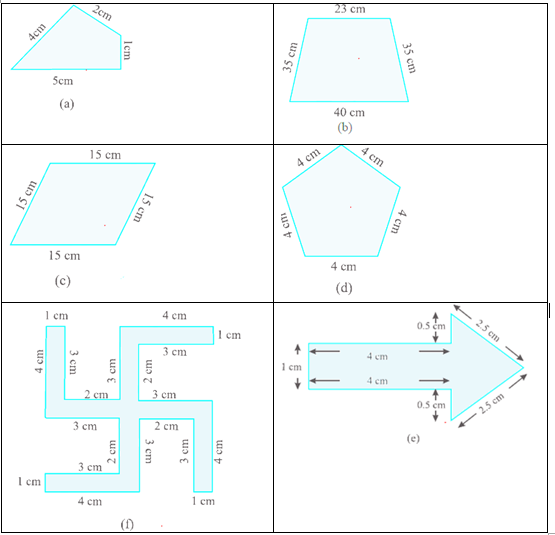Perimeter is the distance covered along the boundary forming a closed figure when you go round the figure once
Or
Perimeter is the sum of the sides of the polygon
(a)
Perimeter = $4 + 2 + 1 + 5 = 12$ cm
(b)
Perimeter = $35 + 23 + 35 + 40 = 133$ cm
(c)
Perimeter = $15 + 15 + 15 + 15 = 60$ cm
(d)
Perimeter = $4 + 4+ 4 + 4 + 4 = 20$ cm
(e)
Perimeter = $1 + 4 + 0.5 + 2.5 + 2.5 + 0.5 + 4 = 15$ cm
(f)
Perimeter = $4 + 1 + 3 + 2 + 3 + 4 + 1 + 3 + 2 + 3 + 4 + 1 + 3 + 2 + 3 + 4 + 1 + 3 + 2 + 3 = 52$ cm

Question 2
The lid of a rectangular box of sides 40 cm by 10 cm is sealed all round with tape. What is the length of the tape required?
As the lid of the box is sealed around with a tape, we need to find the perimeter of the rectangular box.
Length of the rectangular box = 40 cm
Width of the box = 10 cm
We know that Perimeter of a rectangle is given by
= 2 (length + width)
So, length of tape required = perimeter of the rectangular box = 2 (40 + 10) = 100cm

Question 3
A table-top measures 2 m 25 cm by 1 m 50 cm. What is the perimeter of the table-top?
The important thing in these question is the Unit conversion. We need to either convert m into cm or cm into m. It is good to convert into lowest unit to make it easier
Length of the table-top = 2 m 25 cm = 2 m + 0.25 m = 2.25 m
Width of the table-top = 1 m 50 cm = 1 m + 0.50 m = 1.50 m
We know that Perimeter of a rectangle is given by
= 2 (length + width)
Perimeter = 2(length + width) = $2(2.25 + 1.50) = 2(3.75) = 7.50$ m

Question 4
What is the length of the wooden strip required to frame a photograph of length and breadth 32 cm and 21 cm respectively?
A frame is put around the photograph and hence to find the length of the wooden strip to frame we find the perimeter of the photograph.
Length of the photograph = 32 cm
Width of the photograph = 21 cm
We know that Perimeter of a rectangle is given by
= 2 (length + width)
Perimeter = 2(length + width) =$2(32 + 21) = 2(53) = 106$cm

Question 5
A rectangular piece of land measures 0.7 km by 0.5 km. Each side is to be fenced with 4 rows of wires. What is the length of the wire needed?
Length of the rectangular piece of land = 0.7 km
Width of the land = 0.5 km
The rectangular piece of land is to be fenced all around with wire. Hence, perimeter of the rectangular land = length of the wire.
We know that Perimeter of a rectangle is given by
= 2 (length + width)
Perimeter = 2(length + width) =$2(0.7 + 0.5) = 2(1.2) = 2.4$ km
Each side is to be fenced with 4 rows of wires. So total length of the wire needed is 4 times the perimeter.
Total length of wire needed = 4(2.4) = 9.6 km

Question 6
Find the perimeter of each of the following shapes:
(a) A triangle of sides 3 cm, 4 cm and 5 cm.
(b) An equilateral triangle of side 9 cm.
(c) An isosceles triangle with equal sides 8 cm each and third side 6 cm.
a) Perimeter = $(3 + 4 + 5 ) = 12$ cm
b) An equilateral triangle is a triangle whose sides are equal.
Given, side of an equilateral triangle = 9 cm
Perimeter = 9 cm + 9 cm + 9 cm OR 3 × 9 = 27 cm
c) An isosceles triangle is a triangle with two equal sides.
Given, equal sides = 8 cm and third side = 6 cm
Perimeter = 8 cm + 8 cm + 6 cm OR (2 × 8) + 6 cm = 22 cm

Question 7
Find the perimeter of a triangle with sides measuring 10 cm, 14 cm and 15 cm.
Perimeter of a triangle = $10 cm + 14 cm + 15 cm = 39 cm$

Question 8
Find the perimeter of a regular hexagon with each side measuring 8 m.
A regular hexagon has six sides which are equal.
Given the measure of a side of a hexagon = 8 m
So, Perimeter of the hexagon = 6 × 8 = 48 m

Question 9
Find the side of the square whose perimeter is 20 m.
Perimeter of a square is given by = 4 × side of a square
Given Perimeter = 20 m
So, 20 m = 4 × side
Side of the square = 20 / 4 = 5 m

Question 10
The perimeter of a regular pentagon is 100 cm. How long is its each side?
A regular pentagon has five sides which are equal.
So, perimeter of a regular hexagon = 5 × side.
Given the perimeter of a regular hexagon = 100 cm
So, 100 cm = 5 × side
Side = 100 / 5 = 20 cm

Question 11
A piece of string is 30 cm long. What will be the length of each side if the string is used to form:
(a) a square?
(b) an equilateral triangle?
(c) a regular hexagon?
The string length is the perimeter of the shape in the three options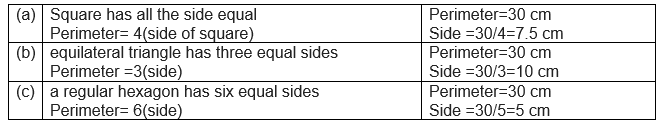Question 12
Two sides of a triangle are 12 cm and 14 cm. The perimeter of the triangle is 36 cm. What is its third side?
Perimeter of the triangle = 36 cm
Sides of the triangle = 12 cm and 14 cm
We know that the perimeter of a triangle = sum of all the sides.
36 cm = 12 cm + 14 cm + third side
36 = 26 + third side
Third side = 36 – 26 = 10 cm
So third side of the triangle is 10 cm

Question 13
Find the cost of fencing a square park of side 250 m at the rate of Rs 20 per metre.
Side of the square = 250m
The square park is to be fenced all around and hence we need to find the perimeter of the park, i.e. length of fencing required = perimeter of the square.
Perimeter of a square = 4 × side = 4 × 250 = 1000 m
So, Length of fencing required = 1000 m
Now, cost of fencing per meter = Rs. 20
So, cost of fencing 1000 m = 1000 × 20 = Rs. 20,000

Question 14
Find the cost of fencing a rectangular park of length 175 m and breadth 125 m at the rate of Rs 12 per metre.
Length of the rectangular park is 175 m
Width of the rectangular park is 125 m
Perimeter of the park = 2(length + width) = $2(175 + 125) = 2(300) = 600$ m
Cost of fencing per meter = Rs. 12
Cost of fencing the park is = 600 × 12 = Rs. 7200

Question 15
Sweety runs around a square park of side 75 m. Bulbul runs around a rectangular park with length 60 m and breadth 45 m. Who covers less distance?
Sweety and Bulbul run around the park and hence the distance covered by them is the perimeter of the park.
Sweety runs around a square park of side 75 m.
Perimeter of the park = $4 \times side = 4 \times 75 = 300$ m
Bulbul runs around a rectangular park of length 60 m and width 45 m
Perimeter of the rectangular park = $2(length + width) = 2(60 + 45) = 2(105) = 210$ m
So, Distance covered by Sweety is 300 m and by Bulbul is 210 m.
So  Bulbul covers lesser distance.

Question 16
What is the perimeter of each of the following figures? What do you infer from the answers?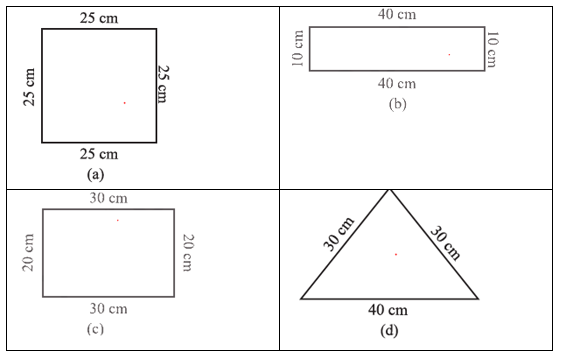(a) Perimeter = $25 + 25 + 25 + 25 = 4 \times 25 = 100$ cm
(b) Perimeter = $20 + 30 + 20 + 30 = 2(20 + 30) = 2(50) = 100$ cm
(c) Perimeter = $10 + 40 + 10 + 40 = 2(10 + 40) = 2(50) = 100$ cm
(d) Perimeter = $30 + 30 + 40 = 100$ cm
Inference: The perimeters of the shapes are all equal.

Question 17
Avneet buys 9 square paving slabs, each with a side of ½ m. He lays them in the form of a square.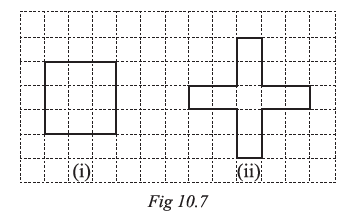(a) What is the perimeter of his arrangement [figure (i)]?
(b) Shari does not like his arrangement. She gets him to lay them out like a cross. What is the perimeter of her arrangement [figure (ii)]?
(c) Which has greater perimeter?
(d) Avneet wonders if there is a way of getting an even greater perimeter. Can you find a way of doing this? (The paving slabs must meet along complete edges i.e. they cannot be broken.)
(a)
The side of each square is ½ m and hence the length of the side of the square formed is
$\frac {1}{2} + \frac {1}{2} + \frac {1}{2} = \frac {3}{2}$ m
So, Perimeter of the square formed is 4 × 3/2 = 4 × 3/2 = 12/2 = 6 m
(b) Shari arranges into cross form
So, the Perimeter of this shape = $\frac {1}{2} + 1 + 1 + \frac {1}{2} + 1 + 1 + \frac {1}{2} + 1 + 1 + \frac {1}{2} + 1 + 1 = 10$ m
(c) It is apparent that Shari’s arrangement has a greater perimeter.
(d)  No, it cannot be arranged such that the perimeter of the image is greater than 10 cm.

## Try These NCERT Book solutions

1. Meera went to a park 150 m long and 80 m wide. She took one complete round on its boundary. What is the distance covered by her?
Distance = perimeter of the park = 2(150 + 80)= 460 m

2. Find the perimeters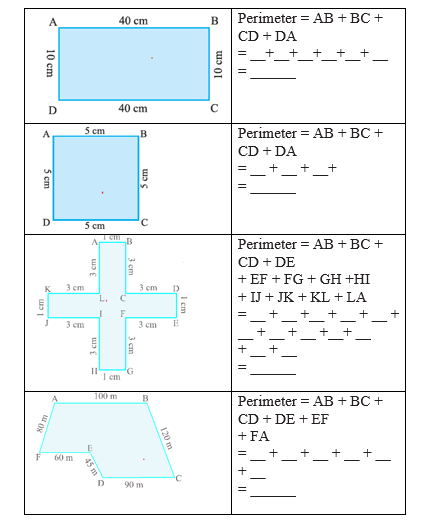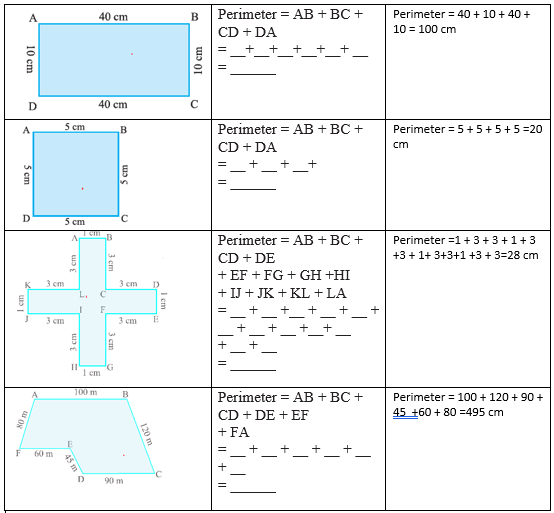### Practice Question

Question 1 What is $\frac {1}{2} + \frac {3}{4}$ ?
A)$\frac {5}{4}$
B)$\frac {1}{4}$
C)$1$
D)$\frac {4}{5}$
Question 2 Pinhole camera produces an ?
A)An erect and small image
B)an Inverted and small image
C)An inverted and enlarged image
D)None of the above